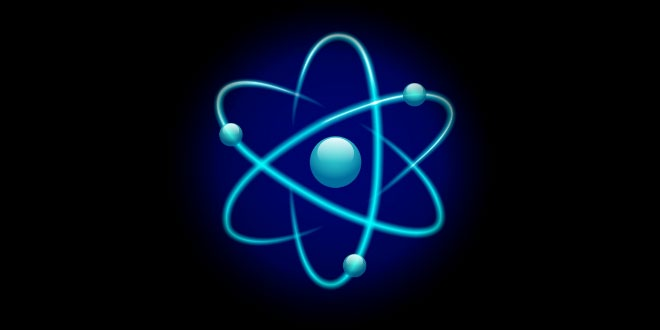Tuesday , July 5 2022# 9th Class (CBSE) Science: Atoms and Molecules

#### Question: Define law of conservation of mass.

Answer: In a chemical reaction mass can neither be created nor destroyed.
E.g., 2Na + Cl2 —–> 2NaCl
2 x 23 + 2 x 35.5 ——> 2(23 + 35.5)

#### Question: Explain law of constant proportion.

Answer: In a chemical substance the elements are always present in definite proportions by mass.
E.g., In water, the ratio of the mass of hydrogen to the mass of oxygen H:O is always 1:8

#### Question: Name the scientist who gave:

1. Law of conservation of mass.
2. Law of constant proportions.

1. Antoine Lavoisier
2. Joseph Proust

Hg → Mercury
Au → Gold
Ag → Silver
Sn → Tin

#### Question: What is meant by automicity? Explain with 2 eg.

Answer: The number of atoms present in one molecule of an element of is called its automicity.

Eg:- Suphur = 8
Ozone = 3

#### Question: Who coined the term atom?

Answer: John Dalton coined the term atomt

#### Question: Define atom.

Answer: The smallest particle of matter, which can take part in a chemical reaction is called atom.

#### Question: Define molecule.

Answer: The smallest particle of an element or compound which can exist independently is called molecule.

#### Question: Define atomicity.

Answer: The number of atoms constituting a molecule is known as its atomicity.

#### Question: What is atomic mass unit?

Answer: The sum of the atomic masses of all the atoms in a molecule of the substance is expressed.in atomic mass unit. E.g., H20 = 1 x 2 + 16 = 18 amu

#### Question: How do atoms exist?

Answer: Atoms exist in the form of atom, molecule or ions.

#### Question: Give the atomicity of phosphorous and nitrogen.

Answer: The atomicity of phosphorus is P4 i.e., 4.
The atomicity of nitrogen is N2 i.e., 2.

#### Question: What is an ion?

Answer: Charged atom is called as an ion. The ion can be positively charged called cation or negatively charged called anion.

Anion => Cl

#### Question: Give one difference between cation and anion.

Answer: Cations are positively charged ion.
Anions are negatively charged ion.

#### Question: Give the chemical formula for ammonium sulphate.

NH4+ SO42-
Chemical formula —-> (NH4)2S04.

#### Question: What is Avogadro’s constant?

Answer: The Avogadro’s constant (6.022 x 1023) is defined as the number of atoms that are present in exactly 12 g of carbon-12.

= (2 x 1) + (16)
= 2 + 16 = 18 u

#### Question: Give the unit to measure size of atom and give size of hydrogen atom.

Answer: The unit to measure size of atom, is nanometer, size of hydrogen atom is 10-10m.

#### Question: What is IUPAC, give its one function?

Answer: IUPAC is International Union for Pure and Applied Chemistry. It approves the names of elements.

#### Question: Give the Latin name for sodium, potassium, gold and mercury.

Answer: Sodium —> Natrium, Gold —> Aurum
Potassium —> Kalium, Mercury —> Hydrargyrum

#### Question: What is the ratio by mass of combining elements in H20, C02 and NH3?

Answer: H2O ratio by mass of combining elements 2 : 16 —>1 : 8 (H : O)
C02 ratio by mass of combining elements 12 : 32—> 3 : 08 (C : O)
NH3 ratio by mass of combining elements 14 : 3—>14 : 3 (N : H)

#### Question: Define valency and give the valency for the following elements: Magnesium, Aluminium, Chlorine and Copper.

Answer: Valency: The combining capacity of an element is called its valency.

Valency of the following elements:
Magnesium – 2
Aluminium – 3
Chlorine – 1
Copper – 2

#### Question: What is polyatomic ton? Give one example.

Answer: A group of atoms carrying a charge is known as a polyatomic ion.
E.g., Ammonium – NH4+
Nitrate – N03

#### Question: Write down the formula for: Copper nitrate, calcium sulphate and aluminium hydroxide.

Copper nitrate —> Cu(N03)
Calcium sulphate —> CaS04 Aluminium hydroxide Al(OH)3

#### Question: It is possible to see atoms these days? Explain.

Answer: The Size of atom is so small that it cannot be seen by naked eye or by simple optical instruments.
To see atoms of different elements a special type of microscope called Scanning Tunneling microscope (STM) is used.

#### Question: Write the formula of the following compounds. Water, Ammoniua, Methane, Sulphur Dioxide, Ethanol.

Water                         →        H2 O                     →          Hydrogen & Oxygen
Ammoniua               →        NH3                     →          Nitrogen & Hydrogen
Methane                    →       CH4                           →          Carbon & Hydrogen
Sulphur Dioxide    →       SO2                           →          Sulphur & Oxygen
Ethanol                      →       C2H5OH              →          Carbon & Hydrogen and Oxygen

#### Question: What is formula unit mass? How is it different from molecular mass?

Answer: The formula unit mass of a substance is a sum of the atomic masses of all atoms in a formula unit of a compound. The constituent particles of formula unit mass are ions and the constituent particles of molecular mass are atoms.

## Sources of Energy: 10th Science Chapter 14

Class: 10th Class Subject: Science Chapter: Chapter 14: Sources of Energy Quiz: – Questions MCQs: …